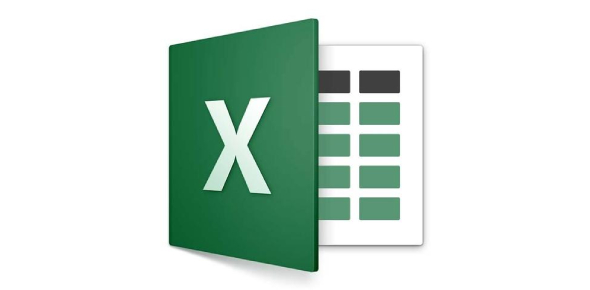# Test Your MS Excel Basics: Quiz! Trivia

20 Questions | Total Attempts: 2916SettingsReady to test your MS Excel Basics? Here is a quick quiz for you. Do you think you will Ace this quiz? This quiz involves your knowledge of the easiest way to add up columns of numbers, change the column width to fit the contents, add a new row, and the correct sequence to follow for a user to remove a spreadsheet from a workbook. It will all add up if you take this quiz.

• 1.
A fast way to add up this column of numbers is to click in the cell below the numbers and then:
• A.

Click Subtotals on the Data menu.

• B.

View the sum in the formula bar.

• C.

Click the AutoSum button on the Standard toolbar, then press ENTER.

• 2.
Say that you want to paste a formula result — but not the underlying formula — to another cell. You would copy the cell with the formula, then place the insertion point in the cell you want to copy to. What next?
• A.

Click the Paste button on the Standard toolbar.

• B.

Click the arrow on the Paste button on the Standard toolbar, then click Formulas.

• C.

Click the arrow on the Paste button on the Standard toolbar, then click Values.

• 3.
How do you change the column width to fit the contents?
• A.

Single-click the boundary to the left of the column heading.

• B.

Double-click the boundary to the right of the column heading.

• C.

Press ALT and single-click anywhere in the column.

• 4.
There are three worksheets with every new workbook. You can change that automatic number if you want to.
• A.

True

• B.

False

• 5.
###### means:
• A.

You've entered a number wrong.

• B.

You've misspelled something.

• C.

The cell is not wide enough.

• 6.
To add a new row, click a cell in the row immediately above where you want the new row.
• A.

True

• B.

False

• 7.
Which key do you press to group two or more nonadjacent worksheets?
• A.

CTRL.

• B.

SHIFT

• C.

ALT

• 8.
To copy an entire worksheet and all its data, you should click the worksheet tab of the sheet that you want to copy, hold down SHIFT, and then drag the selected sheet along the row of sheet tabs.
• A.

True

• B.

False

• 9.
A user wishes to remove a spreadsheet from a workbook. Which is the correct sequence of events that will do this?
• A.

Go to FILE - SAVE AS - SAVE AS TYPE - Excel 4.0 Work Sheet

• B.

Right click on the spreadsheet tab and select DELETE

• C.

Right click on the spreadsheet and select INSERT - ENTIRE COLUMN

• 10.
Which formula can add the all the numeric values in a range of cells, ignoring those which are not numeric, and place the result in a different cell?
• A.

Count

• B.

Average

• C.

Sum

• 11.
Is it possible to insert an image from a file into an Excel spreadsheet ?
• A.

Yes

• B.

No

• 12.
Can an Excel spreadsheet be used as the "data source" for a Word Mail Merge?
• A.

Yes

• B.

No

• 13.
On an Excel sheet the active cell is indicated by ____.
• A.

A dark wide border

• B.

A dotted border

• C.

• D.

None of the above

• 14.
A Formula and a function are the same thing.
• A.

True

• B.

False

• 15.
In order to multiply items in Excel you would use:
• A.

^

• B.

@

• C.

*

• D.

#

• 16.
The formula = ((A2+B5)*5% is valid.
• A.

True

• B.

False

• 17.
If cells: A1=90 A2=85 A3=80 A4=75 A5=75 What will be your formula if you are going to get the average?
• 18.
If cells: A1=90 A2=85 A3=80 A4=75 A5=75 What will be your formula if you are going to get the total?
• 19.
Get the remarks in cell E10 that valued 75 which noted, if the remarks will be higher than 74, the remarks will be "PASSED" or else "FAILED".
• 20.
Get the remarks in cell A1 that valued 75 which noted, if the remarks will be higher than 74, the remarks will be "PASSED" or else "FAILED".
Related TopicsBack to top